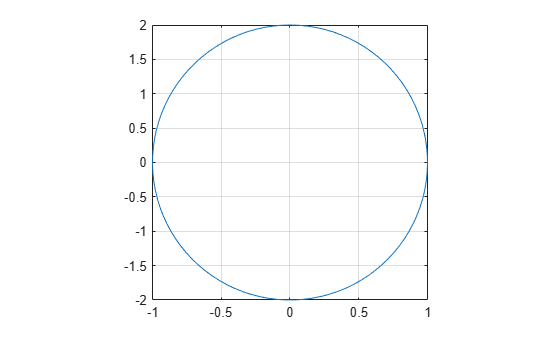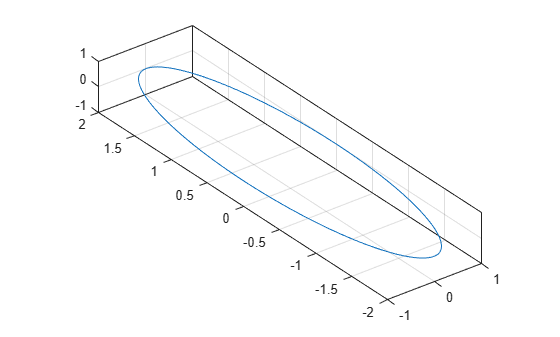Documentation

Control Ratio of Axis Lengths and Data Unit Lengths

You can control the relative lengths of the x-axis, y-axis, and z-axis (plot box aspect ratio). You also can control the relative lengths of one data unit along each axis (data aspect ratio).

Plot Box Aspect Ratio

The plot box aspect ratio is the relative lengths of the x-axis, y-axis, and z-axis. By default, the plot box aspect ratio is based on the size of the figure. You can change the aspect ratio using the pbaspect function. Set the ratio as a three-element vector of positive values that represent the relative axis lengths.

For example, plot an elongated circle. Then set the plot box aspect ratio so that the x-axis is twice the length of the y-axis and z-axis (not shown).

t = linspace(0,2*pi);
plot(sin(t),2*cos(t))
grid on
pbaspect([2 1 1])Show the axes in a 3-D view to see the z-axis.

view(3)For square axes, use [1 1 1]. This value is similar to using the axis square command.

t = linspace(0,2*pi);
plot(sin(t),2*cos(t))
grid on
pbaspect([1 1 1])Data Aspect Ratio

The data aspect ratio is the relative length of the data units along the x-axis, y-axis, and z-axis. You can change the aspect ratio using the daspect function. Set the ratio as a three-element vector of positive values that represent the relative lengths of data units along each axis.

For example, set the ratio so that the length from 0 to 1 along the x-axis is equal to the length from 0 to 0.5 along the y-axis and 0 to 2 along the z-axis (not shown).

t = linspace(0,2*pi);
plot(sin(t),2*cos(t))
grid on
daspect([1 0.5 2])Show the axes in a 3-D view to see the z-axis.

view(3)For equal data units in all directions, use [1 1 1]. This value is similar to using the axis equal command. One data unit in the x direction is the same length as one data unit in the y and z directions.

t = linspace(0,2*pi);
plot(sin(t),2*cos(t))
grid on
daspect([1 1 1])Revert Back to Default Ratios

Change the data aspect ratio. Then revert back to the default plot box and data aspect ratios using the axis normal command.

t = linspace(0,2*pi);
plot(sin(t),2*cos(t))
grid on
daspect([1 1 1])
axis normal